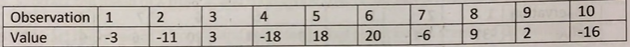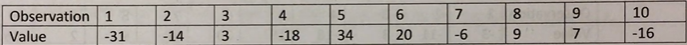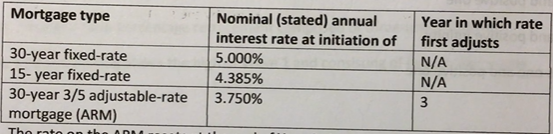## Free CFA Level 1 Practice Test 51 Questions CFA Mock Exam Answer Keys AMBIPi

Hi CFA Aspirants, welcome to AKVTutorials. Are you preparing for CFA Level 1, 2, 3 exams for making a career in CFA (Charted Financial Analyst). According to CFA Wikipedia, CFA The Chartered Financial Analyst (CFA) program is a postgraduate professional certification offered internationally by the American-based CFA Institute. A candidate who successfully completes the program and meets other professional requirements is awarded the “CFA charter” and becomes a “CFA charter holder”. Therefore, you need CFA Study Notes and Free CFA Level 1 Practice Test 51 Questions CFA Mock Exam Answer
Keys AMBIPi

In this article, you will get Free CFA Level 1 Mock Exam Practice Questions.

## Free CFA Level 1 Mock Practice Exam Questions Bank

Free CFA Level 1 Practice Question No: 511:

The following 10 observations are a sample drawn from an approximately normal population:The sample standard deviation is closest to:

Option A : 13.18

Option B : 12.50

Option C : 11.92

Option A: 13.18

CFA Level 1 Exam Question No: 512:

The following ten observations are a sample drawn from an approximately normal  population:The sample standard deviation is closest to:

Option A: 17.56

Option B: 18.58

Option C: 19.59

Option C : 19.59

Free CFA Level 1 Mock Exam Question No: 513:

Which of the following most accurately describes how to standardize a random variable X?

Option A: Subtract the mean of X from X, and then divide that result by the standard deviation of Х.

Option B: Subtract the mean of X from X, and then divide that result by the standard deviation of the standard normal distribution

Option C: Divide x by the difference between the standard deviation of X and the standard deviation of the standard normal distribution

Option A : Subtract the mean of X from X, and then divide that result by the standard deviation of Х.

CFA Level 1 Free Practice Question No: 514:

A descriptive measure of a population characteristic is best described as a:

Option A: parameter

Option B: frequency distribution

Option C: sample statistic

Option A : parameter

Free CFA Practice Question No: 515:

In generating an estimate of a population parameter, a larger sample size is most likely to improve the estimator’s:

Option A: consistency

Option B: efficiency

Option C: unbiasedness

Option C : consistency

CFA Level 1 Sample Question No: 516:

The probability of Event A is 40%. The probability of Event В is 60%. The joint probability of AB is 40%. The probability (P) that A or В occurs, or both occur, is closest to:

Option A: 60%

Option B: 40%

Option C: 84%

Option A : 60%

Free CFA Level 1 Quiz Question NO: 517:

A U.S. Treasury bill (T-bill) has 90 days to maturity and a bank discount yield of 3.25%. The effective annual yield (EAY) for the T-bill is closest to:

Option A: 3.29%

Option B: 3.32%

Option C: 3.36%

Option C : 3.36%

Free CFA Level 1 Quiz Question NO: 518:

A borrower is considering three competing mortgage loan offers from her bank. The amount borrowed on the mortgage is \$100,000 with monthly compounding.The rate on the ARM resets at the end of Year 3. Assuming the ARM is reset at 5.500% (i.e., the remaining balance on the loan will now be repaid with 5.500% nominal annual interest), which of the three loans will have the smallest monthly payment after the rate reset at the end of Year 3?

Option A: 30-year ARM

Option B: 15-year fixed-rate loan

Option C: 30-year fixed-rate loan

Option C : 30-year fixed-rate loan

Free CFA Practice Question No: 519:

By definition, the probability of any event, E, is a number between:

Option A: zero and positive one

Option B: zero and positive infinity

Option C: minus one and positive one

Option A : zero and positive one

CFA Mock Exam Free Question No: 520:

In setting the confidence interval for the population mean of a normal or approximately normal distribution and given that the sample size is small, Student’s t-distribution is the preferred approach when the variance is:

Option A: large

Option B: known

Option C: unknown